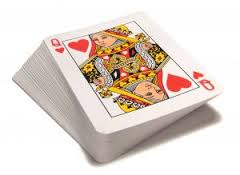# A probability problem by Trung Le

Probability Level 1

A standard 52-card deck is divided into two unequal piles. Given that the probability of drawing a face card from the smaller pile is 4/11, and the probability of drawing a face card from the bigger pile is 2/15, find the number of face cards in the smaller pile.×